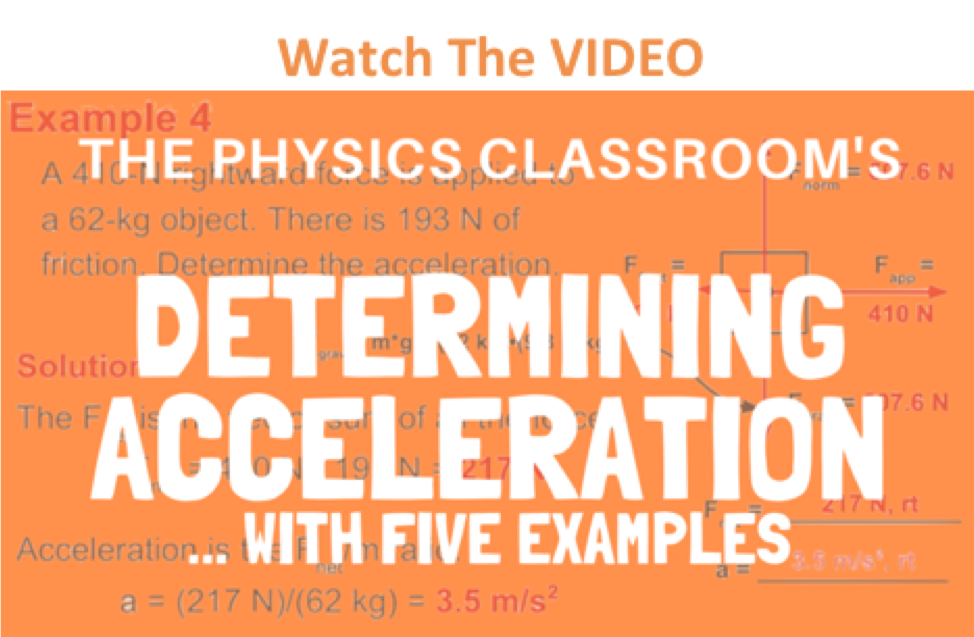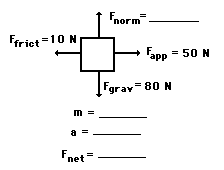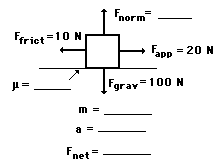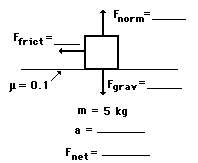Newton's Laws - Lesson 3 - Newton's Second Law of Motion

# Finding AccelerationAs learned earlier in Lesson 3 (as well as in Lesson 2), the net force is the vector sum of all the individual forces. In Lesson 2, we learned how to determine the net force if the magnitudes of all the individual forces are known. In this lesson, we will learn how to determine the acceleration of an object if the magnitudes of all the individual forces are known. The three major equations that will be useful are the equation for net force (Fnet = m•a), the equation for gravitational force (Fgrav = m•g), and the equation for frictional force (Ffrict = μ • Fnorm).

The process of determining the acceleration of an object demands that the mass and the net force are known. If mass (m) and net force (Fnet) are known, then the acceleration is determined by use of the equation.

a = Fnet / m

Thus, the task involves using the above equations, the given information, and your understanding of Newton's laws to determine the acceleration. To gain a feel for how this method is applied, try the following practice problems. Once you have solved the problems, click the button to check your answers.

### Practice #1

An applied force of 50 N is used to accelerate an object to the right across a frictional surface. The object encounters 10 N of friction. Use the diagram to determine the normal force, the net force, the mass, and the acceleration of the object. (Neglect air resistance.)### Practice #2

An applied force of 20 N is used to accelerate an object to the right across a frictional surface. The object encounters 10 N of friction. Use the diagram to determine the normal force, the net force, the coefficient of friction (μ) between the object and the surface, the mass, and the acceleration of the object. (Neglect air resistance.)### Practice #3

A 5-kg object is sliding to the right and encountering a friction force that slows it down. The coefficient of friction (μ) between the object and the surface is 0.1. Determine the force of gravity, the normal force, the force of friction, the net force, and the acceleration. (Neglect air resistance.)A couple more practice problems are provided below. You should make an effort to solve as many problems as you can without the assistance of notes, solutions, teachers, and other students. Commit yourself to individually solving the problems. In the meantime, an important caution is worth mentioning:

Avoid forcing a problem into the form of a previously solved problem. Problems in physics will seldom look the same. Instead of solving problems by rote or by mimicry of a previously solved problem, utilize your conceptual understanding of Newton's laws to work towards solutions to problems. Use your understanding of weight and mass to find the m or the Fgrav in a problem. Use your conceptual understanding of net force (vector sum of all the forces) to find the value of Fnet or the value of an individual force. Do not divorce the solving of physics problems from your understanding of physics concepts. If you are unable to solve physics problems like those above, it is does not necessarily mean that you are having math difficulties. It is likely that you are having a physics concepts difficulty.

### We Would Like to Suggest ...Sometimes it isn't enough to just read about it. You have to interact with it! And that's exactly what you do when you use one of The Physics Classroom's Interactives. We would like to suggest that you combine the reading of this page with the use of our Force Interactive. You can find it in the Physics Interactives section of our website. The Force Interactive allows a learner to explore the effect of variations in applied force, net force, mass, and friction upon the acceleration of an object.

Visit:  Force

1. Edwardo applies a 4.25-N rightward force to a 0.765-kg book to accelerate it across a tabletop. The coefficient of friction between the book and the tabletop is 0.410. Determine the acceleration of the book.

2. In a physics lab, Kate and Rob use a hanging mass and pulley system to exert a 2.45 N rightward force on a 0.500-kg cart to accelerate it across a low-friction track. If the total resistance force to the motion of the cart is 0.72 N, then what is the cart's acceleration?

Next Section: